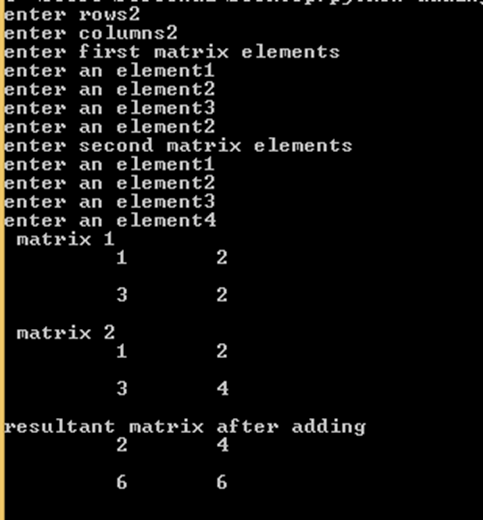Here you will get program for python matrix addition. In python “list” concept is there rather than arrays.  For implementing matrix we should take help of lists in python. Matrix can be represented as nested lists.

Suppose if we want to implement 2×3 matrix then python syntax will look like this.

Matrix=[[1,2,3],[4,5,6]]     #This is 2×3 matrix

If we want to implement 3×2 matrix then

Matrix=[[1,2],[3,4],[5,6]]     #This is 3×2 matrix.